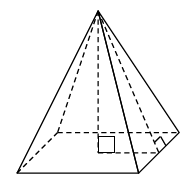Home > CCG > Chapter 11 > Lesson 11.1.3 > Problem11-40

11-40.Find the volume and surface area of a square-based right pyramid if the base edge has length 6 units and the height of the pyramid is $4$ units. Assume the diagram at right is not to scale.

$\text{Volume }= \frac{1}{3} (\text{volume of the corresponding prism})$

$\frac{1}{3} (\text{number of cubes in the bottom layer)(number of layers)}$

$\frac{1}{3}(l)(w)(h)$

$\frac{1}{3}(6^2\cdot4)$

$\text{Surface Area} = 4\text{(area of triangle) + (area of base)}$
You will need to find the slant height of the pyramid.

$96$ square units